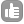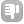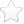1What defines the area of effect of nuclear magnetic resonance? Is it the magnetic field? the frequency? Or just the diameter of the coils? asked Feb 17 '11 at 00:29Hello, it is not very clear what exactly you are asking about - by area of effect - do you mean the active area of the sample? Please explain a bit. Thanks. - Evgeny Fadeev (Feb 17 '11 at 10:59)1just for the fun, you can do nmr with earth field... some ground topology analysis use nmr at earth field, so the sample is at kilometer scale :) answered Feb 22 '11 at 10:02Yoan Monneau 1361NMR is a 3 dimensional action, and so occurs in a volume rather than over an area. The active volume of an NMR Spectrometer is equal to the length of the probe's RF coil multiplied by the cross-sectional area of the inside of the sample tube. answered Feb 22 '11 at 10:040The question is not clear but if you mean the sample, the rf frequency on the sample you can see this here: http://qa.nmrwiki.org/question/38/what-is-the-optimal-sample-length-with-shigemi-tubes answered Feb 21 '11 at 02:420Field is proportional to frequency, so it has the same "areas" (whatever you mean by "area"). Diameter of coils effects on sensitivity because you have more sample producing the signal of free induction decay. answered Mar 22 '11 at 19:00